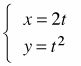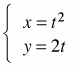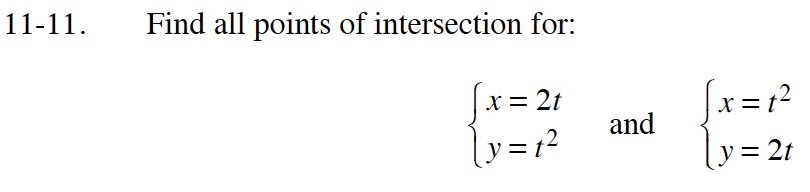### Home > CALC > Chapter 11 > Lesson 11.1.1 > Problem11-11

11-11.

Find all points of intersection for: Homework Help ✎andWhat do you notice about these sets of equations?

These are inverse functions. Where do the graphs of inverse functions intersect?

Inverse functions intersect where x = y.
Therefore, solve 2t = t2.

Note: This solves for t, not the points of intersection.